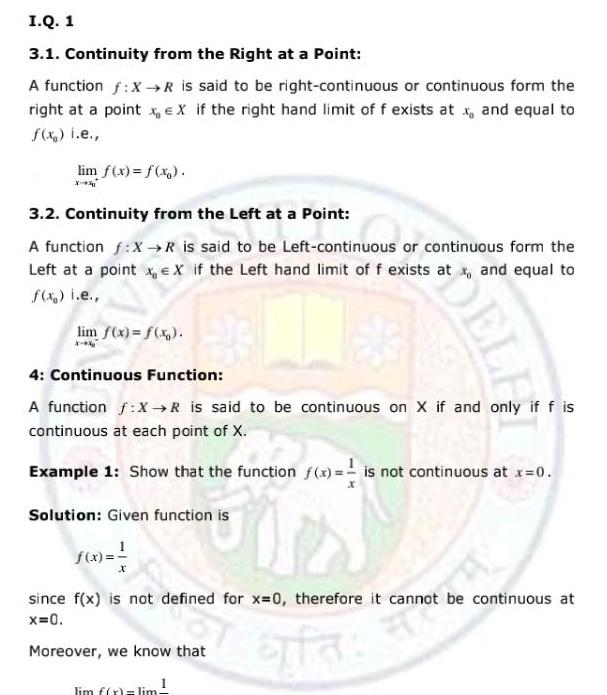Question:

# I Q 1 3 1 Continuity from the Right at a Point A function f

Last updated: 9/14/2023I Q 1 3 1 Continuity from the Right at a Point A function f X R is said to be right continuous or continuous form the right at a point x EX if the right hand limit of f exists at x and equal to f x i e lim f x f x X X 3 2 Continuity from the Left at a Point A function f X R is said to be Left continuous or continuous form the Left at a point x EX if the Left hand limit of f exists at x and equal to f x i e lim f x f x x k DELL 4 Continuous Function A function f X R is said to be continuous on X if and only if f is continuous at each point of X Example 1 Show that the function f x is not continuous at x 0 J Solution Given function is f x 1 since f x is not defined for x 0 therefore it cannot be continuous at x 0 Moreover we know that fa lim f x lim